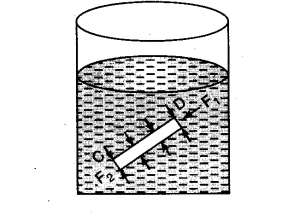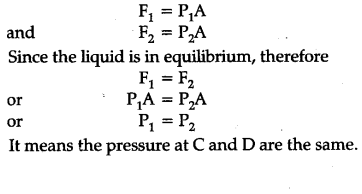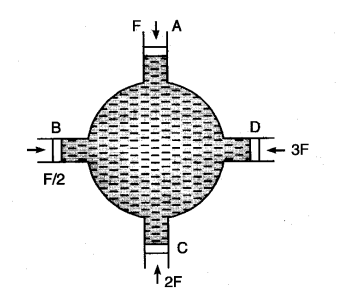# State and prove Pascal's law and give its experimental proof also

It states that if gravity effect is neglected, the pressure at every point of liquid in equilibrium of rest is same.
Proof : Consider two points C and D inside the liquid in a container which is in equilibrium of rest. Imagine a right circular cylinder with axis CD of uniform cross-sectional area A such that points C and D lie on float faces of the cylinder in figureThe liquid inside the cylinder is in equilibrium under the action of forces exerted by the liquid outside the cylinder. These forces are acting every where perpendicular to the surface of the cylinder. Thus force on the flat faces of the cylinder at C and D will perpendicular to the forces on the curved surface of the cylinder. Since the liquid is in equilibrium, therefore, the sum of forces acting on the curved surface of the cylinder must be zero. If P1 and P2 are the pressure at points C and D and F1 and F2 are the forces acting on the flat faces of the cylinder due to liquid,Experimental Proof. Consider a spherical vessel having for cylinderical tubes A, B, C and D each fitted with air tight frictioriless piston of area of corss-section a, a/2, 2a and 3a respectively.
Fill the vessel with an incompressible liquid so that no air gap is left inside the vessel and piston fitted in the various cylindrical tubes. Push the piston A with force F.
It is seen that all other pistons will be pushed outwards. To keep the pistons at their respective original positions, the force F/2, 2F and 3F respectively required to be applied on pistons of tubes B, C and D respectively to hold them. Now pressure developed on liquid in tubes, B, C and D are F/2, 2F/2a, 3F/3a i.e., such,equal to F/a. This indicates that the pressure applied is transmitted equally to all parts of liquid. This proves Pascal law